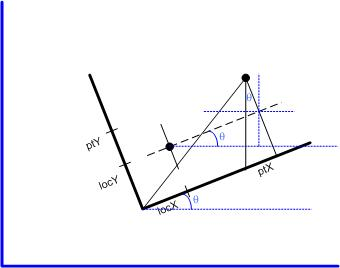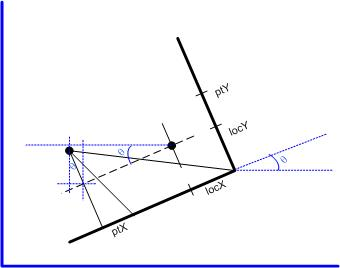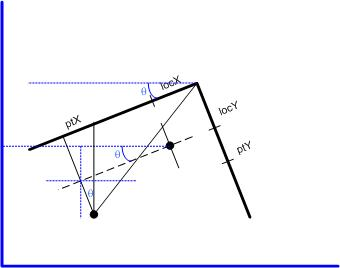Coordinate Transformations In Visio

Everybody does coordinate transformations with translation and rotation. There is nothing too challenging there. Microsoft Visio, takes it a bit further, though, and includes translation, rotation, and flipping (mirroring) around the local x/y axes. When working on a recent project in Visio, we needed to find the global page coordinates, given the local shapesheet coordinates. This required a little bit of thought into the coordinate transformations that Visio must use.

The figure below shows the situation. The local coordinate system (in black) is rotated (angle theta) around the point (locX, locY) in local coordinates. Visio provides the point of rotation also in page coordinates, as (PinX, PinY). The point of interest is given in local coordinates as (ptX, ptY). (This might be Connection point 1, Controls.Row_1, etc.)The trick is that the ShapeSheet also contains the properties FlipX and FlipY. If FlipX is TRUE and FlipY is FALSE, then you get a scenario like:If FlipX is FALSE and FlipY is TRUE, then:IIf FlipX and FlipY are both TRUE, then:Fortunately, inspection of the graphs above, shows the normal coordinate rotation matrix can be easily altered in the presence of flipping.Where (capital) X and Y are the point of interest in page coordinates, (lower case) x and y are the point of interest in local coordinates (given in the ShapeSheet), l_x is LocPinX, l_y is LocPinY (the point of rotation in local coordinates), theta is the rotation of the local coordinate system (Angle in the ShapeSheet), and PinX and PinY are the point of rotation in the page coordinates. f_x is 1 if FlipX=FALSE, and -1 if FlipX=TRUE, and similar for f_y.

No big deal, but I doubt that I will remember this if I don't write it down.